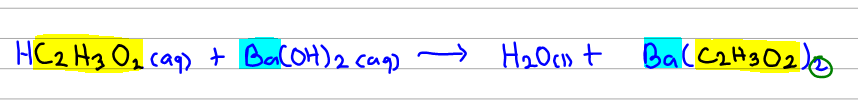# Problem: Consider the unbalanced equation for the neutralization of acetic acid:HC2H3O2(aq) + Ba(OH)2(aq) → H2O(l) + Ba(C2H3O2)2(aq)Balance the equation.

###### FREE Expert Solution

To balance the reaction easily we can look at C2H3Olike a polyatomic ion. This means that we balance it separately like its a single particle instead of breaking it down to its elements and balancing those elements individually.In the reaction above, I've highlighted parts in blue and yellow. Ba here seems to be balanced for now so everything with Ba we leave alone (as in we don't change the coefficient for now).

90% (323 ratings)###### Problem Details

Consider the unbalanced equation for the neutralization of acetic acid:

HC2H3O2(aq) + Ba(OH)2(aq) → H2O(l) + Ba(C2H3O2)2(aq)

Balance the equation.